# Dictionary

Click on the appropriate letter to be taken to the required section.

 A B C D E F G H I J K L M N O P Q R S T UV WXYZ

 Letter aaaa Word aaaa Description A alloy A mixture of metals added together in molten form. An alloy has physical properties quite different to the component metals. Alloys tend to have much higher electrical resistances than the metals that compose them. The purer a metal the lower its electrical resistance. alternating current (A.C) An electric current where the charge moves back and forth in a wave pattern (the charges oscillate or alternate their motion in the conductors). The charges flow first in one direction and then reverse and move back in the opposite direction. The terminals of an A.C power supply change their polarity, swapping from being positive to negative and back again at a certain frequency, usually 50 Hz in Australia (i.e. 50 times per second). ampere The scientific unit for electric current, symbol A. ONE ampere is equivalent to a flow of electric charge equal to one coulomb of charge per second, i.e. I = Δq/Δt , where I is the electric current in ampere, q is the charge in coulombs, and t is the time in seconds. Ampere (Andre-Marie)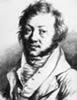French scientist who was a pioneer in the study of electricity and magnetism. He showed that electricity and magnetism were linked and began studies on electromagnetism and electrodynamics. The unit for electric current is named after him. He also developed a mathematical equation to calculate the magnetic force acting between two current carrying wires. This relationship is known as Ampere's Law. atom Fundamental building blocks of normal matter. Name comes from the ancient Greek "atomis" meaning indivisible. First discussed by Ancient Greek philosopher, Democritus. Today we know that all atoms have a nucleus containing protons (positively charged particles) and neutrons (neutral particles) except the smallest atom - hydrogen. The nucleus is orbited by the very tiny negatively charged particles called electrons. In a neutral (uncharged atom) the number of protons in the nucleus is equal to the number of orbiting electrons. The number of protons in the nucleus determines what element the atom is. Protons and neutrons are almost the same mass while the electrons are very tiny in comparison. As such the nucleus of the atom contains nearly all the mass. B battery (of cells) A number (group) of electrochemical cells attached together. Supplies direct current (D.C) when attached in an electric circuit. It has become common for people to refer to a single electrochemical cell as a "battery". (see Electrochemical cell) brine A solution of common salt (sodium chloride), e.g. ocean sometimes referred to as the 'briney deep'. Contains ions that allow the brine solution to conduct electricity. C cell(electric) A single electrochemical cell producing a DC voltage to supply electrical energy. Commonly called a "battery". Consists of TWO different conductors (electrodes) with an electrolyte (conductive solution) between them. In a normal dry cell, a carbon rod and a zinc can (the electrodes) with a conductive paste between them (the electrolyte). The cell relies on the electrochemical difference of the two electrodes to produce a D.C voltage between them. charge Related to being electric, the charge on an object is normally due to an excess or deficiency of electrons. Negative charge - excess electrons, Positive charge - electrons have been removed leaving more protons compared to electrons. chemical bonds The bonds that form between atoms to hold them together in matter structures. These bonds involve the valence electrons and are influenced by the positive charge on the nucleus of the atoms involved. circuit A number of electrical components attached to each other via conducting leads. Generally contains, a power source to supply a potential difference (voltage) to the circuit; conducting leads to allow current to flow around the circuit, a switch to turn the electric current on or off, i.e. to make or break the circuit; a load that uses the electrical energy to do something, e.g. a light globe converts the electrical energy into light and heat energy, an electric motor converts the electrical energy into mechanical (kinetic) energy. circuit breaker A safety device that behaves like a fuse to prevent excessive current flowing into a circuit which may cause overheating and an electrical fire. Generally found in large boxes or small rooms. The external power cable from the supply runs first to a circuit breaker. Most circuit breakers operate on an electromagnetic effect where, when the current is too large, an electromagnet attracts a spring loaded switch which breaks the circuit and cuts the power supply. The switch must be re-set before any current can flow. close-pack crystal lattice A characteristic of metallic elements whereby the atoms pack very close to each other within the crystal lattice. This leads to the outer electron orbits basically overlapping which gives rise to most metals being very good conductors of electricity and heat. coal-fired powerstation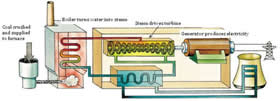A power station that burns coal to produce the energy needed to generate electricity. Most power stations in Australia are coal-fired. compounds A pure substance composed of two or more elements chemically combined. Definition: Two or more different elements chemically combined together in fixed and definite proportions. A compound cannot be simplified by physical means but can be broken into the component elements using chemical processes. conductor A material that allows the movement of electric charge through it. The best conductors are "superconductors" that offer NO resistance to charge moving through them but, they must be cooled to extremely low temperatures to become a superconductor. Pure silver, gold and copper are the best conductors that offer the LOWEST resistance to charge moving through them. Most metal alloys conduct electricity but offer some resistance to the movement of charge through them. The purer a metal is the lower its electrical resistance. conservation of energy A fundamental scientific principle which has been found true in ALL areas of study. "Energy can neither be created nor destroyed but only rearranged in form." This means that forms of energy can be changed from one form to another but you can never get out more energy than was in the system to begin. Energy losses from systems usually mean that, when energy is transformed from one form to another, you usually end up with LESS energy than was present at the start. conventional current The flow of positive charge. Currents were being studied long before the structure of an atom was known. Currents were believed to flow be flow of positive charge which left the POSITIVE terminal of the power supply to travel around the circuit and arrive back to the NEGATIVE terminal. Science now knows that negative electrons really flow in metal wires but conventional current is still used when studying electricity. coulomb Symbol 'Q' or'q'. The scientific unit for electric charge. The electric charge arises because of the natural electric charge born by the components within ALL atoms, i.e. positive protons in the nucleus, and negative electrons in orbit. Note that it takes about 6.25 x 1018 electron or proton charges to equal a charge of 1.0 coulomb (that is 6 250 000 000 000 000 000 ). This means that when a current of 1.0 ampere is flowing in a circuit, that many electrons are flowing past a point in the circuit each second, i.e. 1 ampere = 1 coulomb of charge per second. Named after the scientist Augustin de Coulomb who did many early studies on electricity. crystal lattice The regular arrangement of the atoms within a solid that results in a crystal form. All solids are crystalline but generally the crystals are not large enough to be easily seen. current Symbol I - A movement of electric charge in a conducting media, e.g. a metal wire. Measured in ampere (Symbol: A). Equivalent to the number of coulombs of charge per second flowing passed a point. Given by mathematical equation - I = Δq/Δt D de Coulomb (Charles Augustin)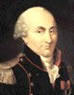French scientist and mathematician who developed Coulomb's Law which describes the size of the forces acting between two charged objects. He developed the very sensitive "torsion balance" to allow him to collect accurate results. The equation below shows Coulomb's Law.density The mass per unit volume measure in kg.m-3. Related to the total number of atoms and the sum of their individual masses found within a certain volume. Density calculates by dividing mass of an object by the volume of the object. direct current (DC) An electric current that flows in just ONE direction through a circuit. A current (conventional) from a DC power supply is considered to flow from the positive terminal of the power supply, around the circuit to arrive back at the negative terminal of the DC power supply. Note that we now know it is electrons which flow in currents. E Edison (Thomas)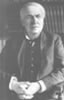American inventor who came to fame for his invention of the first light globe that would last for a long time. Founded a company that began supplying electricity to cities. Developed a D.C system with DC motors and lights. Involved in the so called "Battle of the Currents" with George Westinghouse who wanted to use an A.C system to supply electricity. efficiency The efficiency of any machine is a measure of how well the device can convert energy from one form to another. The efficiency of a machine can be determined by dividing the OUTPUT ENERGY by the INPUT ENERGY and multiplying by 100. electric field A region of influence which surrounds ALL electric charges. The direction of the field shows the direction that a 'test positive charge' would experience a force if placed in the field. The electric field gets weaker quickly as you move further away from the charged object. electrical device Any device which use electrical energy to operate. An electrical device converts the electrical energy into another form. The device uses energy from the electric current flowing it to operate. electricity The name given to a form of energy that involves electric charges in motion. The voltage gives energy to the charges while the current flowing converts the electrical energy into a different form. The voltage can be considered a form of potential energy, while the current flowing in the wire is a form of kinetic energy. electrochemical An electrochemical device relies on the chemical difference between two different materials. This difference is due to the structure of the different atoms involved. The higher the electrochemical activity of an element the more violently it will react in a chemical reaction. (Refer to electrochemical series of metals (Google) electrode Name sometimes used to describe a source of electric charge, e.g. the positive terminal of a battery could be termed the 'positive electrode' electrolyte Any time an electric charge moves, it produces a magnetic field. By coiling conducting wire around an iron core and then passing a D.C current through the coil, an electromagnet is formed. The magnetic field is only produced while the current is flowing. The direction of the magnetic field depends on the direction of the current in the coils of the electromagnet. electromagnetic induction If any conducting material experiences a changing magnetic field it will induce a voltage in the conductor. This principle is employed in generators where a coil of wire moves through a magnetic field. electromagnetic radiation Form of energy consisting of waves of electric and magnetic field that travel through a vacuum at the speed of light (300 00 km.s-1.). Visible light is an electromagnetic radiation, also includes, radio waves, microwaves, infrared, ultraviolet, X-rays, and Gamma radiation. Energy of electromagnetic radiation carried as 'photons' and the total energy related to; The intensity of the beam (how many photons) and, the frequency of the wave (energy of photon). Energy of a photon given by, E = h f, where 'h' is Planck's constant. electron The negatively charged particle found in ALL atoms. The electrons are extremely tiny particles and orbit at relatively large distances from the nucleus of the atom. Lightest component in atoms with a mass of 9.11 x 10-31 kg and an electric charge of - 1.6 x 10-19 coulombs. electrostatic Electric charge which remains stationary e.g. trapped on an insulator or on an insulated conductor. Objects which have an electrostatic charge produce an electric field that will create forces on any object which comes close enough to it. element A pure substance consisting of atoms of one kind only. They cannot be broken down by physical or chemical means into any simpler substance. energy Energy is defined as "the ability to work". (Unit: joules, symbol J). Obeys the "Law of Conservation of energy" which states," Energy can neither be created nor destroyed but only rearranged in form." There are Two main forms of energy "potential" (stored) and "kinetic" (moving). In an electric circuit the battery supplies potential energy to charges while a current represents charges with kinetic energy. F Faraday (Michael)British scientist who discovered many important details on electrical energy and performed many significant experiments. He was the first to produce an electric motor and discovered electromagnetic induction which led him to design the first generator. A true experimental genius who determined many important details on electricity and magnetism proving them to be intimately linked. Franklin (Benjamin)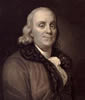Early American scientist and politician. Defined positive and negative charge using Glass rubbed with silk (Glass - positive) and Ebonite rubbed with fur (wool) (Ebonite - negative). First to show that the small sparks being studied in the laboratory were the same (just very much smaller) than the lightning strikes observed in the sky. This is the famous experiment where Franklin flew a specially constructed kite during a lightning storm. (This is NOT to be recommended). fossil fuel A fuel that has formed from once living organisms that have died, been buried, and over time have formed a fuel. The energy trapped in all fossil fuels came from the sunlight that was present when the organism was alive, e.g. coal, oil, and natural gas. Fossil fuels take many millions of years to form. fuel cell A very efficient form of D.C electric cell that operates with a chemical reaction which consumes a fuel (the anode side [positive]) with an oxidiser (the cathode side [negative]), e.g. the hydrogen fuel cell turns the fuel, hydrogen, into water by combining the hydrogen with oxygen. There are wide array of different fuel cells. fuse A safety device included in a circuit that is designed to melt, breaking the circuit, IF excessive current flows through it. A fuse prevents equipment attached to the circuit from being damaged and help to prevent electrical fires. G generator A device to produce electrical energy by electromagnetic induction. Generally involves a coil of wire which is rotated in a magnetic field. Any time that a conductor experiences a changing magnetic field (by moving through a magnetic field) then a voltage will be induced in the conductor. Work is done to rotate the coil of a generator and this energy is converted into kinetic energy of the spinning coil. As the coil moves through the magnetic field, the charges in the conductor that makes up the coil experience an electrical potential energy difference (a voltage) due their motion through the magnetic field. This allows a generator to supply electrical energy to an external circuit as long as the coil is kept spinning and continues to move through the magnetic field. geothermal energyHeat energy from the Earth, (geos - Earth, thermal - heat). An enormous of heat energy is trapped below the surface of the Earth. Geothermal energy involves piping water into fractured HOT rock and then collecting the pressurised hot water (steam) and using it to drive a turbine to operate a generator. global warming The phenomenon where evidence suggests the Earth is slowly increasing in temperature due to certain greenhouse gases increasing in concentration in the Earth's atmosphere, particularly CO2. These greenhouse gases are mainly the result of human activities (particularly by burning fossil fuels). The increasing greenhouse gases in the atmosphere are reducing the amount of heat lost from the Earth to space. graphite Pure form of carbon which is a fairly good electrical conductor, e.g. the lead in a pencil is made of graphite. greenhouse gas 'Gases in the Earth's atmosphere that at night absorb heat radiating away from the surface of Earth, acting like a blanket and reducing the amount of cooling. Water vapour and carbon dioxide are important greenhouse gases. Methane is a powerful greenhouse gas and about 20 times more heat absorbing than carbon dioxide. The increase in greenhouse gases produced by human activity has resulted in an enhanced greenhouse effect and is causing Global warming. gravitational potential energy The energy possessed by a mass due to its location in the gravitational field of another massive object. The gravitational potential energy of an object is defined as "the work done to move an object from a very distant point (infinity) to a position in the gravitational field of another massive object." Because gravity will act to do work to try to move masses closer together, this means that gravitational potential energy, a scalar quantity, always has a negative value because WORK would need to be done to move the object to INFINITY to allow it to escape the gravitational field of the massive object. When starting relatively close to each other, the gravitational potential energy of a body due to the gravitational field of another massive object will INCREASE as the distance between the masses increases. Unit: joule (J). (See Equations sheet for detail) gravity The interaction which acts to create forces between objects with MASS. Gravity always creates an ATTRACTIVE force between masses. One of the FOUR (now 3) fundamental forces with the gravitational force mediated by graviton. Considered the weakest of the natural forces but the one that now has the most influence on the structural features making up the observed Universe. H heat A form of energy that results from the total kinetic energy of the particles in a sample. The higher the temperature the more kinetic energy particles possess, i.e. Ek(av) = 3/2 k T , where k is Boltzmann's constant. hydroelectric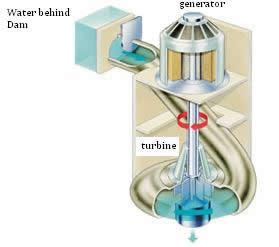A system that uses moving water, usually stored behind dams, to turn a turbine that operates a generator to produce electricity. I ion An electrically charged atom or groups of atoms. The charge on the ion is due to the removal or addition of electrons. If an atom (group of atoms) loses a negatively charged electron, it will have a positive charge, i.e. it is a positive ion. If electrons are added to an atom or group of atoms then it ends up a negatively charged ion. ionic bond A chemical bond, usually between a metal and non-metal that involves the metal atom losing electrons to the non-metal atom. This produces a positively charged metal ion and a negatively charged non-metal ion. The two ions are attracted to each other and form a crystalline solid termed a SALT. Many ionic compounds are soluble in water which results in an ionic solution that will conduct electricity (usually with a high resistance). ionised The removal or addition of electrons from particles. This results in the particle becoming electrically charged. instantaneous At an "instant in time" - the instantaneous value of a quantity is referring to its value at a particular instant in time. insulator (electrical) A material that restricts or retards the movement of charge through it. In a good insulator the electrons are bound very strongly in the material and require a very large amount of energy (very high voltage) to be released to flow as a current, e.g. plastics. Heat insulation - restricts or retards the movement of heat energy through them. insulation A material with a very high electrical resistance which does not normally conduct electricity. In an insulator the electrons are bound very strongly and require large amounts of electrical energy to be released, e.g. plastics, dry air. J joule (J) The standard unit for WORK and ENERGY. Symbol J. Defined as the work done on a 1 kg mass to accelerate it at 1 metre per second squared for each metre travelled. Named after English scientist James Prescott Joule who proved experimentally the equivalence of mechanical work and heat energy gained. Joule (James Prescott)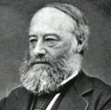An English physicist and brewer who proved experimentally the equivalence of mechanical work and heat. His work led to the law of conservation of energy and the First Law of thermodynamics. His first scientific paper involved an explanation of the heating of a wire in an electric circuit. He placed a wire coil in water and measured the change in temperature of the water when a known current was passed through the wire for a certain time. He was able to show that the electrical energy lost by the wire was equal to the heat energy gained by water. The standard S.I. unit for energy is named after him. K kinetic energy The energy possessed by a body due to its MOTION. The kinetic energy can be calculated using the equation; Ek = 1/2 m v2 , where 'm' is the mass of the object in kilograms and 'v' is the velocity in ms-1. (Ek Units: joules.) L lattice A regular arrangement of the structural components. In matter, the lattice of component atoms gives rise to a crystalline structure when solid. Law of Conservation of Energy The law which states “Energy can neither be created nor destroyed BUT ONLY rearranged in form". Includes mass-energy from E = m c2. A very important law which has been tested and found to be true every time energy is involved. light Visible to human eye, light is a form of electromagnetic radiation with wavelengths from about 400 nm (red) to 700 nm (violet), (1 nm = 10-9 m ). Travels in a vacuum at 'c' (300 000 km per second) as fast as anything known to science (proposed as "terminal velocity" by Einstein). Energy of light carried as photons where the energy of the photon is given by E = h f , where h is Planck's constant. M magnetic field A region of influence produced around any moving electric charge. All atoms produce magnetic fields due to the electrons and protons but normally these fields tend to cancel each other out. When a current flows in a wire a nett magnetic field is produced around the wire. By coiling the current carrying wire around an iron core (cylinder of iron) an electromagnet is produced. Some materials are naturally magnetic, e.g. the iron mineral known as magnetite. Magnet surveys can reveal important information on structures, particularly if they contain iron. magnets A material that produces a magnetic field around it. Permanent magnets have an internal structure where the internal magnetic fields are lined up to add with each other. This results in the final material being a magnet and producing an observable magnetic field. molecule An element where the atoms have the following properties; All form positively charged ions by losing electrons to form salts. Can form alloys when molten metals are mixed together, conduct heat and electricity well, Tend to have high melting point and are solid at room temperature (except mercury), Tend to be malleable (able to be beaten into sheets) and ductile (able to be drawn into wires), and  Form close-pack cubic or hexagonal crystals. motor (electric) An electrical device which converts electrical energy into mechanical energy (kinetic energy). Operates when current flowing through the coils of the motor interact with a magnetic field and experience a torque (turning force) and begin to rotate. Both AC and DC motors exist. metal A combination of two or more non-metal atoms chemically combined together in fixed proportions held together mainly by covalent bonding as the non-metal atoms share valence electrons. N negative Form of electric charge found on the electron that is a component of all atoms. An object with a negative charge contains an EXCESS of negative electrons compared to the positive protons found in the nuclei. An object with a negative charge will repel any other electrons that come within its region of influence. neutron Neutral particles found in the nucleus of all atoms except hydrogen. Mass almost the same as the proton (tiny bit heavier). Involved in creating nuclear interactions that keep the protons and neutrons contained in the tiny, dense nucleus of atoms. Newton's FIRST Law of motion "An object will remain at rest, or travelling at a constant velocity, UNLESS acted upon by a net, unbalanced, external force." Known as the Law of INERTIA and describing the conditions necessary for an "inertial" frame of reference. Newton's SECOND Law of motion "The rate of change of a body’s momentum is directly proportional to the applied net force." This law describes the idea of IMPULSE. It is generally simplified to say; "for a certain mass, the acceleration is directly proportional to the applied net force", i.e. F = m a. Newton's THIRD Law of motion "To every action there is an equal and opposite reaction". This is the best known law but is often misunderstood. It simply states that "if a force from something else acts on an object, the object's inertia acts to try to create an equal force in the opposite direction to the force applied to it". This action/reaction pair involves TWO things. non-metal Elements that are characterised by the following properties; Form negatively charged ions by gaining electrons but many also bond covalently by sharing electrons with other non-metal atoms, Found as either solids, liquids, or gases at room temperature. Solids usually have low melting point (diamond (pure carbon) is an exception), Brittle when solid, and Poor conductors of heat and electricity (insulators). nuclear powerstation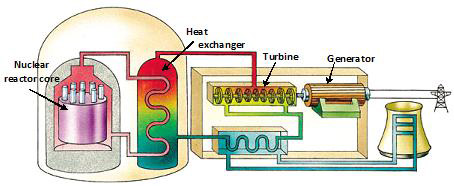A power station that uses nuclear fission of uranium to produce heat and steam to drive a turbine and generator to supply electrical energy. The nuclear fission uses neutrons to split uranium atoms to produce the smaller fission fragments, more neutrons, and lots of heat energy. A controversial form of generating heat to supply electricity due to concerns over radioactive wastes, accidents, or the possibility of creating nuclear weapons. nucleus Very dense, tiny core of all atoms containing protons and neutrons except hydrogen where the nucleus is a single proton. The nucleus contains nearly all of the mass of the atom but the diameter of the nucleus is less than a ten thousandth of the diameter of the atom. O ohm - Ω Unit of electrical resistance. If a voltage of 1 volt is applied to a 1 ohm resistor then a current of 1 ampere will flow through the resistor. Named after Georg Ohm who first determined the relationship between voltage, current and the resistance. Symbol - Ω Ohm (Georg)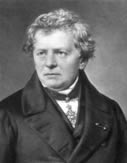German physicist and school teacher who proved experimentally the relationship between the applied potential difference (voltage), the electric current and the resistance. Developed Ohm's law, i.e. V = R I, where V = voltage, I = current and R = resistance. Unit for resistance named after him. Ohm's Law Law determined by Georg Ohm where the resistance of an electrical component can be determined by dividing the voltage for the component by the current flowing through the component, i.e. R = V/I P parallel An electrical connection that allows current to split and flow along different paths. The voltage over parallel resistors is the same and the total resistance of parallel resistors is LESS than the smallest resistor in the circuit. plasma State of matter made of electrically charged particles where electrons have been removed from the positive nuclei so they travel separately, i.e. an ionised gas. Produced at very high temperature more than ten thousand degrees Celsius. Produced in air by lightning strikes and the common form of matter found in stars. positive An electric charge carried on the protons found in the nucleus of all atoms. An object carrying a positive charge has LESS electrons (negative) compared to the positive protons. An object with a positive charge will attract any electrons which come within its region of influence. potential difference (voltage) A measurement of the electrical potential energy difference for a charge at one point compared to another. Measured in volts where 1 volt is equal to a difference in electrical potential energy of 1 joule for each coulomb of charge. Often termed the voltage. potential energy A form of stored energy, e.g. an object raised above the Earth's surface gains gravitational potential energy; the electrons of atoms have chemical potential energy due to the influence of the positive nucleus of the atoms. Electrical potential energy is when a charge at one position can, if a path exists, lose energy by moving to another position. power The rate at which energy is produced or consumed, i.e. Power = Energy/Time, where Power is measured in watts, (symbol W), and a power of 1 watt produces 1 joule of energy per second. The power in an electric circuit can be determined using, P = V I or P = R I2. proton Positively charged particle found in the nucleus of ALL atoms. The mass of a proton is about the same as a neutron but, despite having an equal and opposite charge to the negative electron, the proton is nearly 1900x more massive than an electron. The number of protons in the nucleus determines what element that atom is, e.g. hydrogen - just one proton, carbon - 6 protons, Iron - 26 protons, uranium - 92 protons. Q qualitative A description or measurement which describes a quality of the quantity, e.g. a car can travel "much faster” than a person running. quantitative A description or measurement which includes a quantity e.g. numerical data and units. An example might be, 'A car can travel at up to 30 metres/second while the fastest people can only run at about 10 metres/second.' R rarefied The condition of a gas where it is at a lower pressure and density than air at standard atmospheric pressure. resistance (electrical) The amount of opposition of a material to the flow of electric current through it. Pure metals have the lowest resistance while non-metals tend to have a very high resistance (an insulator). The resistance has to do with how many electrons are able to be mobilised inside the material and how much the electrons interact with nuclei as they move through the material. The higher the temperature the greater the resistance. At very, very low temperatures certain materials become superconductors and offer no resistance to the flow of current. Measured in ohms, symbol Ω resistor A device in an electric circuit where electrical energy is converted into other forms, e.g. heat, as the electrons flowing in the current experience more interactions with the nuclei within the resistor and give up energy to the resistor. The resistance depends on the temperature and the composition of the material that make it up. The resistance is measured in ohms, Ω S semiconductor Class of materials that under the right conditions can act as a conductor and under other conditions as an insulator. Silicon and germanium are natural semiconductors. A semiconductor has a relatively high resistance and only small currents are allowed to flow through them. Semiconductors form the basis of semiconductor theory and an understanding of their behaviour has led to the production of the silicon chip for computers and made a huge contribution to developing technologies. Sometimes termed 'semi-metals' or 'metalloids'. series An electrical connection where current has only one path available and must flow through each component one after the other. The current in a series circuit is the same in all parts and the resistance of a set of series resistance is equal to the sum of the individual resistors in the series circuit. silicon (Si) Element number 14 in the Periodic Table of the elements and the most abundant element on Earth. A natural semiconductor that has allowed the development of the silicon chip and computer technology. Usually found on Earth in silicates (minerals). Extracted from white sands. solar cell A device capable of converting light energy directly into electrical energy. Several forms exist with special doped silicon wafers proving popular and leading to improved efficiency. split-ring commutator Device found in a DC motor or DC generator which provides the attachment to an external circuit to take in current (motor) or provide current to an external circuit (Generator). In a motor the split-ring commutator reverses the current in the rotating coil each half revolution to keep it spinning while, in a generator the split-ring commutator converts the AC being produced by the rotating coil of the generator into DC to supply to the external circuit. static electricity The build up of electric charge on well insulated metal objects OR insulators due to friction or induction, i.e. when two different materials are rubbed against each other or when the object is close to an electrically charged object. It is the production of static electricity that gives rise to the small sparks sometimes experienced when hair is combed. Static electricity can be a large problem when two different materials rub passed each other quickly, e.g. fuel flowing in a fuel pump, an aeroplane travelling through the air. step-down transformer A transformer that is supplied with a higher voltage to the Primary coil and provides a lower voltage from the secondary coil. Only AC can be stepped down with DC not able to be stepped down. step-up transformer A transformer that is supplied with a lower voltage to the Primary coil and provides a higher voltage from the secondary coil. Both AC and DC can be stepped up with a DC step-up transformer often known termed an induction coil. superconductor Materials that offer no electrical resistance to currents. Include some pure metals and metal oxides cooled to a few degrees above absolute zero (-273° Celsius) or a group of ceramics that can become superconductors when cooled by liquid nitrogen (- 196° Celsius). Use is restricted because of the very cold temperatures needed. sustainable An activity where the use of a resource is equal to the rate at which the resource is being naturally replenished and, the use of the resource can be maintained at its present level and the resource will remain available forever. T terminal The attachment points of a power supply, e.g. battery. Also known as electrodes. Tesla (Nikola)Born in the Austrian empire, Tesla was a brilliant Serbian physicist and electrical engineer who was largely responsible for designing the system now used to provide large amounts of electrical energy to sites very distant from the energy source and powerstation. He worked for Edison on improving DC systems and then left to work with George Westinghouse on developing AC systems. Tesla was personally responsible for developing many of the AC components used in present day large scale electricity supply systems. Described by many as "the man who invented the 20th century and shed light over the surface of the Earth". The unit for magnetic field strength, the tesla, is named after him. Made a huge contribution to an understanding of electromagnetism. thermal Referring to HEAT, e.g. thermal energy, thermometer. transformer Electrical device that consists of two coils of wire (Primary and secondary coil) which are magnetically linked by a soft iron core. By using different numbers of loops in the primary coil compared to the secondary coil, the voltage supplied to the primary coil can be 'transformed' into a lower or higher voltage. A transformer relies on changing magnetic fields to work so they operate far more efficiently with AC rather than DC. DC can only be stepped-up to a higher voltage. A step-up transformer produces a higher output voltage from the secondary coil and has less loops of wire in the primary coil compared to secondary coil whereas a step-down transformer produces a lower voltage from the secondary coil and has more loops of wire in the primary coil compared to the secondary coil. turbine A device which use a source of kinetic energy, e.g. steam or water, to make it rotate at high speed. The turbine is attached to a generator and as it spins it rotates the generator which produces electricity. V valence The outermost shell of electrons in orbit around an atom. It is the valence shell of electrons for an atom that determines the physical and chemical properties of an element. volt The standard unit for potential difference (voltage). 1 volt represents an electrical potential energy of 1 joule for each coulomb of charge. Named after Alessandro Volta who produced the first electrochemical cell (battery) which provided the first continuous source of electrical energy. Volta (Alessandro)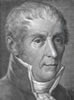An Italian scientist who, about 1800, was responsible for the invention of the simple electrochemical cell and his 'battery' of cells (voltaic pile). Volta discovered that when TWO different metals are connected to each other via an electrolyte (salty solution), when wires were attached to the metals and then brought close to each other, a spark would jump between the wires. He had invented the first electrochemical cell. Volta found that when the cells were stacked on each other (Volta called it a battery of cells) the spark produced got larger and stronger. voltage (potential difference) A common language term referring to the potential difference, i.e. the amount of electrical potential energy per coulomb of charge at one point compared to another. Unit volts (V). W watt The unit of power. 1 watt = 1 joule per second, i.e. the power is the energy delivered divided by the time over which it was delivered, P = E/t . Watt (James)Scottish engineer and inventor who is mainly responsible for the invention and development of the steam engine which was to lead to the industrial revolution. Unit of 'power' named after him. weight The gravitational force created on a body which attracts its mass to the surface of another very massive object (usually Earth). Varies according to the gravity produced by the massive object. The stronger the gravity the GREATER the weight of an object. The weight is equal to the product of; the mass of the object and, the gravitational acceleration caused by the massive object, i.e. on the surface of Earth weight of a body is given by Fg = m g. Unit: newton (N). Westinghouse (George)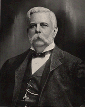American engineer, business man, and entrepreneur who started a company supplying AC systems in competition with Thomas Edison's DC systems. Westinghouse's AC systems won the "battle" due to their ability with AC to use transformers to transfer electrical energy efficiently at very high voltages over long distances and then transform it to lower voltage close to the user for safety. work Work is a scalar quantity measured in joules. The work done on an object is equivalent to the energy gained by the object. Work is done on an object when a force acts to move the object in the direction of the applied force.